## Analogy

#### Analogy

Direction: Each of the following questions consists of two sets of figures. Figures (a), (b), (c) and (d) constitute the Question Set while figures (1), (2), (3), (4) and (5) constitute the Answer Set. There is a definite relationship between figures (a) and (b). Figure (a) and (b) are related in a particular way or manner. Establish the same relationship between (c) and (d).

1. Select a suitable figure from the answer figures that would replace the question mark (?) from question figure.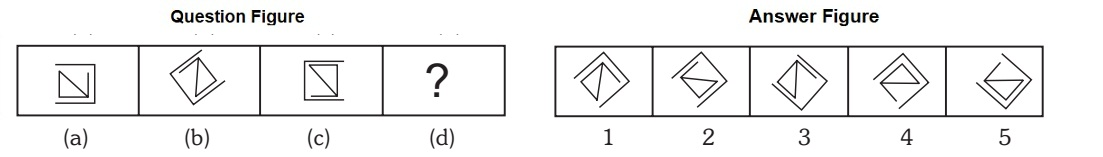1. The outer element rotates 135 degree clock wise and the inner element rotates 135 degree anti clock wise.

##### Correct Option: A

The outer element rotates 135 degree clock wise and the inner element rotates 135 degree anti clock wise.

1. Select a suitable figure from the Answer Figures that would replace the question mark (?) from question figure.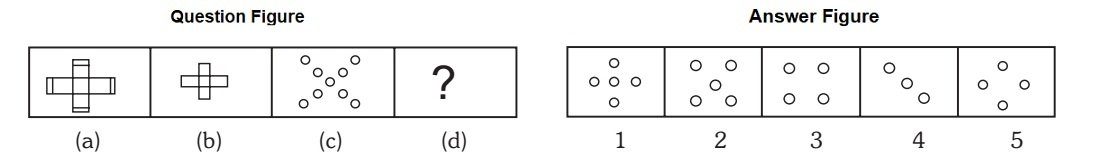1. Four part of the outer end lost.

##### Correct Option: B

Four part of the outer end lost.

1. Select a suitable figure from the Answer Figures that would replace the question mark (?) from question figure.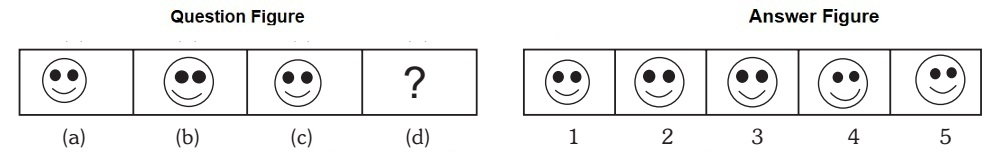1. The two partially shaded circles rotates through 180 degree

##### Correct Option: D

The two partially shaded circles rotates through 180 degree

1. Select a suitable figure from the answer figures that would replace the question mark (?) from question figure.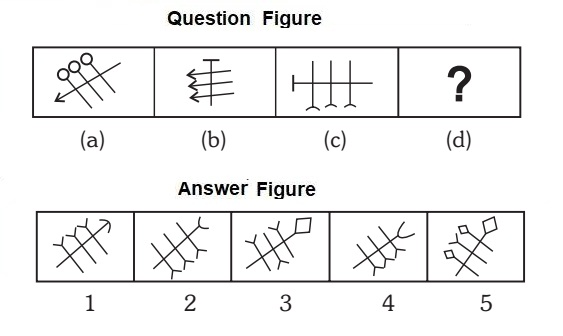1. The three parallel arrows together rotate 45 degree anti – clock wise and the arrow perpendicular to these three arrows rotates 135 degree clock wise. Arrow heads interchange position and colour.

##### Correct Option: D

The three parallel arrows together rotate 45 degree anti – clock wise and the arrow perpendicular to these three arrows rotates 135 degree clock wise. Arrow heads interchange position and colour.

1. Select a suitable figure from the answer figures that would replace the question mark (?) from question figure.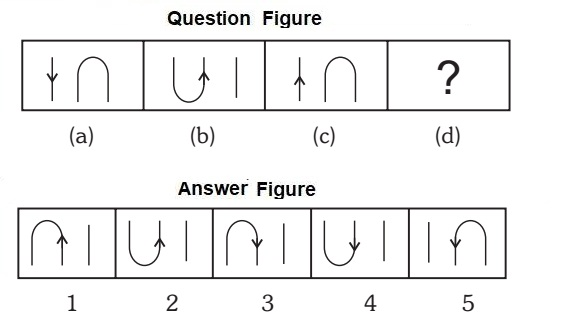1. The complete figure gets rotates through 180 degree and arrow head moves to U – shaped element.

##### Correct Option: C

The complete figure gets rotates through 180 degree and arrow head moves to U – shaped element.